# VBA Wildcards

This tutorial will demonstrate how to use wildcards in VBA.

Wildcards are used in all programming languages and database application like SQL Server. A wildcard can be defined as a symbol that is used to replace one or more characters in a text string.   For example this text string – “mo*” – will find the words mom, mouse, moose, mommy etc; while this text string “mo?” will only find the word mom as the wildcard ? replaces only one character.

We use wildcards with the Like Operator which is an easier alternative to VBA Regex.

## Using the Asterix (*) Wildcard in VBA

The Asterix wildcard replaces one or more characters in a VBA string.

Lets look at the following range of cells in Excel: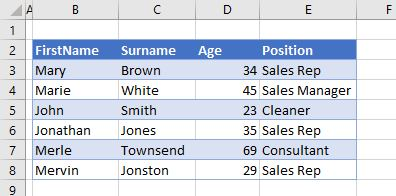By using an Asterix wildcard in our VBA code, we can find all the Firstnames that begin with “M” and change the color of the text to red.

``````Sub CheckForM()
Dim x As Integer
For x = 3 To 8
If Range("B" & x).Value Like "M*" Then
Range("B" & x).Font.Color = vbRed
End If
Next x
End Sub``````

We have therefore looped through the range and found all the first names that begin with the letter M as our wildcard string is “M*

The result of running the code above is shown below.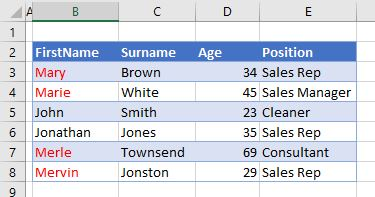If we were to use the wildcard string “Ma*” – then only the first names in B3 and B4 would change.

## Using the Question Mark (?) Wildcard in VBA

The question mark will replace a single character in a VBA string.

Consider the following data: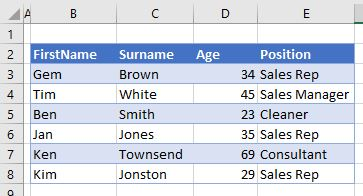We can use the wildcard string “?im” to find any first names that end in “im”

``````Sub CheckForIM()
Dim x As Integer
For x = 3 To 8
If Range("B" & x).Value Like "?im" Then
Range("B" & x).Font.Color = vbRed
End If
Next x
End Sub``````

The result of running this code is shown below: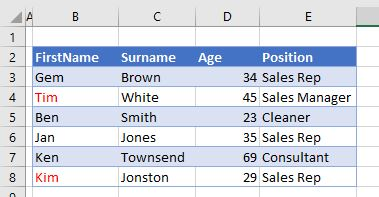## Using [char list]as a Wildcard

The example above can be modified slightly to allow us to use the question mark, in addition to a character list of allowed characters.  The wildcard string can therefore be amended to “?[e-i]m” where the first character can be anything, the second character has to be a character between e and i and the last letter has to be the character “m”.  Only 3 characters are allowed.

``````Sub CharListTest()
Dim x As Integer
For x = 3 To 8
If Range("B" & x).Value Like "?[e-i]m" Then
Range("B" & x).Font.Color = vbRed
End If
Next x
End Sub``````

The result of this code would be:

## Using the hash (#) Wildcard in VBA

The hash (#) wildcard replaces a single digit in a VBA string.   We can match between 0 to 9.

``````Sub CheckForNumber()
Dim x As Integer, y As Integer
For x = 3 To 8
For y = 2 To 5
If ActiveSheet.Cells(x, y) Like "##" Then
ActiveSheet.Cells(x, y).Font.Color = vbRed
End If
Next y
Next x
End Sub``````

The code above will loop through all the cells in the Range (“B3:E8”) and will change the color of the text in a cell to RED if a double-digit number is found in that cell.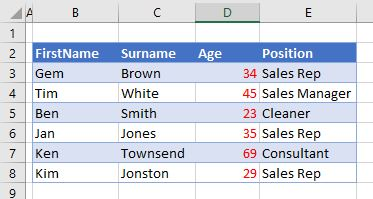In the example below, the code will only change the number if the last number is a 9.

``````Sub CheckFor9()
Dim x As Integer, y As Integer
For x = 3 To 8
For y = 2 To 5
If ActiveSheet.Cells(x, y) Like "#9" Then
ActiveSheet.Cells(x, y).Font.Color = vbRed
End If
Next y
Next x
End Sub``````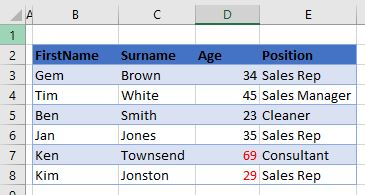Stop searching for VBA code online. Learn more about AutoMacro - A VBA Code Builder that allows beginners to code procedures from scratch with minimal coding knowledge and with many time-saving features for all users!